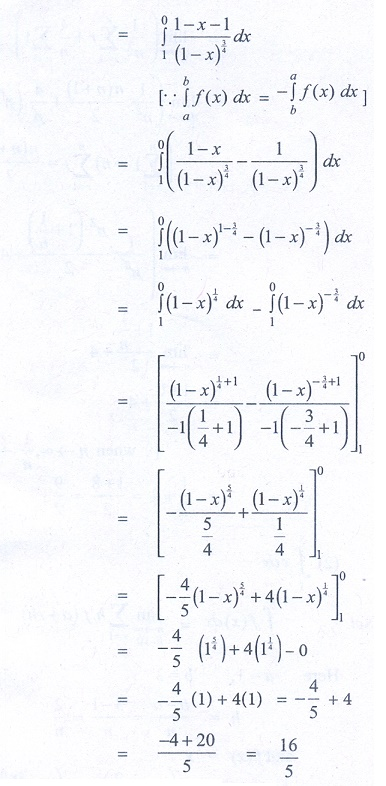Home | | Business Maths 12th Std | Evaluation of Problems using properties of definite integrals

# Evaluation of Problems using properties of definite integrals

Maths: Integral Calculus: Definite integrals: Evaluate the following using properties of definite integrals : Exercise and Example Solved Problems with Answer, Solution

Evaluate the following using properties of definite integrals:

ExampleExercise 2.9

Evaluate the following using properties of definite integrals:1. 0

2. π/2

3. 0

4. π /4

5. 0

6. 16/5

Tags : Exercise and Example Solved Problems with Answer, Solution , 12th Business Maths and Statistics : Chapter 2 : Integral Calculus - I
Study Material, Lecturing Notes, Assignment, Reference, Wiki description explanation, brief detail
12th Business Maths and Statistics : Chapter 2 : Integral Calculus - I : Evaluation of Problems using properties of definite integrals | Exercise and Example Solved Problems with Answer, Solution